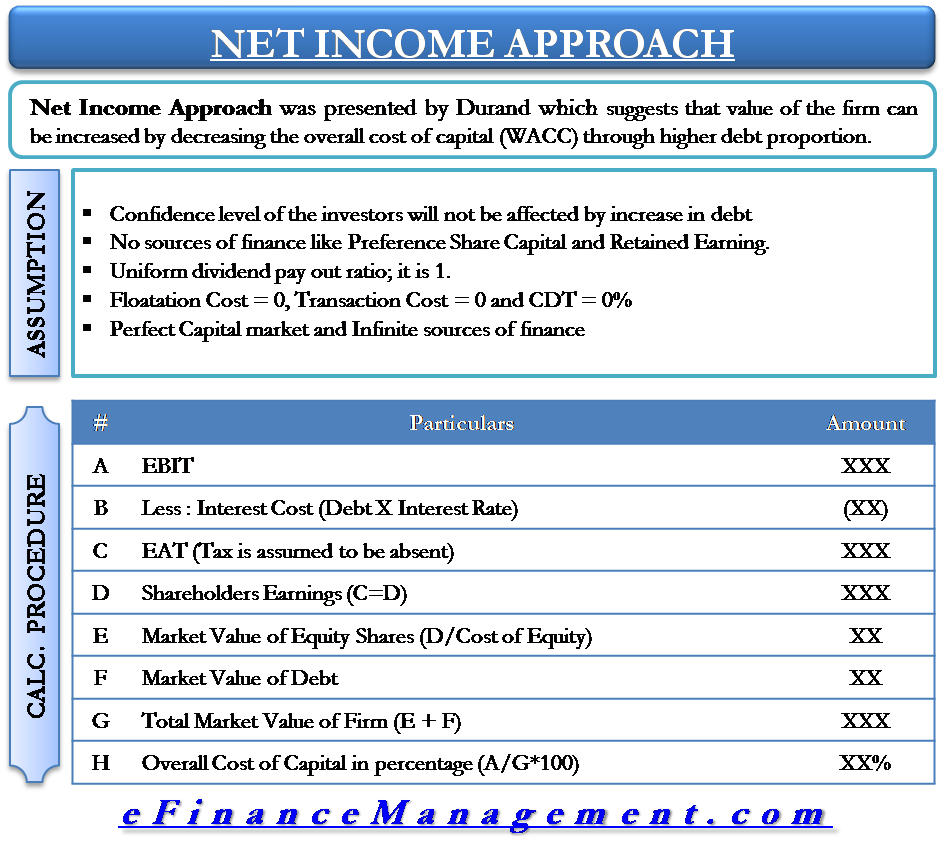# Capital Structure Theory – Net Income Approach

The Net Income Approach suggests that the value of the firm can be increased by decreasing the overall cost of capital (WACC) through a higher debt proportion. There are various theories that propagate the ‘ideal’ capital mix/capital structure for a firm. Capital structure is the proportion of debt and equity in which a corporate finances it’s business. The capital structure of a company/firm plays a very important role in determining the value of a firm.

## Introduction to Capital Structure Theory

A corporate can finance its business mainly by 2 means, i.e., debts and equity. However, the proportion of each of these could vary from business to business. A company can choose to have a structure with 50% each of debt and equity or more of one and less of another. Capital structure is also referred to as financial leverage, which strictly means the proportion of debt or borrowed funds in the financing mix of a company.

Debt structuring can be a handy option because the interest payable on debts is tax-deductible (deductible from net profit before tax). Hence, debt is a cheaper source of finance. But increasing debt has its share of drawbacks like increased risk of bankruptcy, increased fixed interest obligations, etc.
For finding the optimum capital structure to maximize shareholders’ wealth or the value of the firm, different capital structure theories (approaches) have evolved.

Read Capital Structure & its Theories to know more about what is capital structure and what are its different theories. Let us now look at the first approach.

## Net Income Approach Explained

Durand presented the Net Income Approach. The theory suggests increasing the firm’s value by decreasing the overall cost of capital which is measured in terms of the Weighted Average Cost of Capital. This can be done by having a higher proportion of debt, which is a cheaper finance source than equity finance.
Weighted Average Cost of Capital (WACC) is the weighted average costs of equity and debts, where the weights are the amount of capital raised from each source.

 WACC = Required Rate of Return x Amount of Equity + Cost of debt x Amount of Debt Total Amount of Capital (Debt + Equity)

According to Net Income Approach, a change in the financial leverage of a firm will lead to a corresponding change in the Weighted Average Cost of Capital (WACC) and the company’s value. The Net Income Approach suggests that with the increase in leverage (proportion of debt), the WACC decreases, and the firm’s value increases. On the other hand, if there is a decrease in the leverage, the WACC increases, thereby decreasing the firm’s value.

For example, vis-à-vis the equity-debt mix of 50:50, if the equity-debt mix changes to 20: 80, it would positively impact the value of the business and increase the value per share.## Assumptions of Net Income Approach

The Net Income Approach makes certain assumptions which are as follows.

• The increase in debt will not affect the confidence levels of the investors.
• There are only two sources of finance; debt and equity. There are no sources of finance like Preference Share Capital and Retained Earnings.
• All companies have a uniform dividend payout ratio; it is 1.
• There is no flotation cost, no transaction cost, and corporate dividend tax.
• The capital market is perfect; it means information about all companies is available to all investors, and there are no chances of overpricing or underpricing of security. Further, it means that all investors are rational. So, all investors want to maximize their return by minimizing risk.
• All sources of finance are for infinity. There are no redeemable sources of finance.

## Example

Consider a fictitious company with the below figures—all figures in USD.

Calculating the value of a company

Now, assume that the proportion of debt increases from 300,000 to 400,000, and everything else remains the same.

As observed, in the case of the Net Income Approach, with an increase in debt proportion, the total market value of the company increases, and the cost of capital decreases. The reason for this conclusion is the assumption of the NI approach that irrespective of debt financing in capital structure, the cost of equity will remain the same. Further, the cost of debt is always lower than the cost of equity, so with the increase in debt finance, WACC reduces, and the firm’s value increases.

Continue reading Net Income (NI) vs. Net Operating Income (NOI) Approach.Sanjay Borad is the founder & CEO of eFinanceManagement. He is passionate about keeping and making things simple and easy. Running this blog since 2009 and trying to explain "Financial Management Concepts in Layman's Terms".

### 12 thoughts on “Capital Structure Theory – Net Income Approach”

1.Nice explanation. I require more guidance from you.

2.Good
I have understood properly.

3.perfect explanations, keep up

4.brilliant explanation

5.I have a question. Why is this theory named as “NI approach”? I don’t see any reason why this theory is named NI approach. I though from the name the value of a company is related to NI. But the theory does not seem related to NI at all. Please explain to me. Thank you.

6.Should Cost of Debt always be lower than the Cost of Equity? Is it an assumption? Please explain the reason.

•Debt is secured against securities resulting less risk of loss. Less risk brings less returns, hence lesser cost.
On the otherhand risk of loss for the equity holders remains higher and even not secured against any security. Against higer risk equity holders expect higher returns. Hence higher cost of Equity.

7.Very clear easy explanation. Thank you

8.Very clear thank you

9.nice explanations, keep the teaching active

10.•